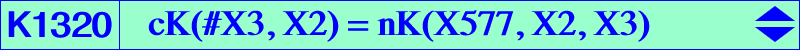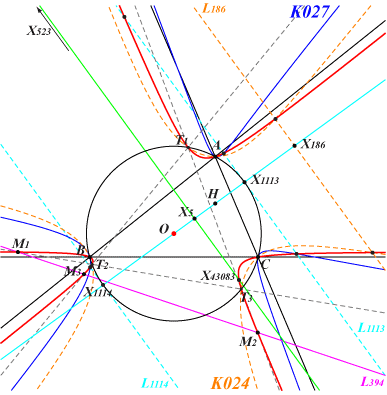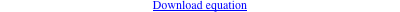too complicated to be written here. Click on the link to download a text file.X(3), X(4240), X(14966), X(15328), X(23981), X(43083), X(52603), X(53232), X(53233), X(53234), X(53235) imaginary foci of the MacBeath inconic vertices of the CircumTangential triangle infinite points of the sidelines of ABC other points below Geometric properties :K1320 is the isogonal transform of K027. It is a nodal cubic with node O and nodal tangents parallel to the asymptotes of the circum-conic with center X(5), perspector X(216). These tangents are real if and only if ABC is obtusangle. The three real symptotes are parallel to the sidelines of ABC and bound a triangle homothetic at X(577) to ABC. K1320 passes through the vertices T1, T2, T3 of the CircumTangential triangle and meets its sidelines again at three collinear points M1, M2, M3 on the trilinear polar of X(394). K1320 is an isogonal cK with respect to this triangle with root X(20). See CircumTangential cubics in Table 25. Hence, K1320 and K024 share six common points on (O) and meet again at three collinear points on the trilinear polar of X(275), passing through X(186), X(523). K1320 and K027 meet at A, B, C, the imaginary foci of the MacBeath inconic and two pairs of points on the perpendiculars at X(1113), X(1114) to the Euler line. The remaining points of K1320 on these lines lie on the tangent at X(110) to (O), passing through X(i) for i = 110, 351, 526, 684, 1576, 1624, 1634, 2421, etc. The tangents at A, B, C meet the respective sidelines of ABC on the trilinear polar of X(577). *** Generalization Let Ω be a point on the Brocard axis. The locus of M whose Ω-isoconjugate (in ABC) and isogonal conjugate with respect to the CircumTangential triangle coincide is a nK with pole Ω, root G, denoted by K(Ω). Hence, K1320 = K(X577). K(Ω) meets the line at infinity on the sidelines of ABC and the three real symptotes are parallel to the sidelines of ABC and bound a triangle homothetic at Ω to ABC. K(Ω) passes through the vertices T1, T2, T3 of the CircumTangential triangle and meets its sidelines again at three collinear points M1, M2, M3 on the line which is the Ω-isoconjugate of (O). This is the trilinear polar of a point Z on the line (GK), hence it is tangent to the Kiepert parabola. The line (ΩZ) is tangent to the inscribed conic with center X(620), perspector X(4590) which is the isotomic conjugate of X(115), also the barycentric square of X(99). This conic is actually the barycentric square of the line (GK). K(Ω) meets K024 share six common points on (O) and meet again at three collinear points on a perpendicular to the Euler line. This is the trilinear polar of the isogonal conjugate of the harmonic conjugate of Ω with respect to O and K. The isogonal conjugate gK(Ω) of K(Ω) is a nK with pole X(32) ÷ Ω, root gΩ. It meets the line at infinity at the same points as K024. It is tritangent to (O) at A, B, C. Obviously, gK(X577) is K027. A remarkable example K(X182) is the unique proper K+ and, at the same time, the unique cubic K0 of this type. Its three asymptotes are the parallels at X(182) to the sidelines of ABC and it meets the sidelines of T1T2T3 again on the trilinear polar of X(183). It meets K024 again on the perpendicular at X(6) to the Euler line. Its isogonal transform is the equilateral cubic nK0(X263, X262).